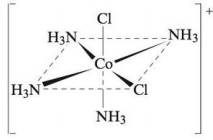Chapter 21, Problem 33E

Chapter
Section
Textbook Problem

# Name the following complex ions:a.b.c.(a)

Interpretation Introduction

Interpretation: The name of the given structures of complex ion is to be stated.

Concept introduction: Rules followed in the naming of coordination compound:

• In naming of coordination compound, the name of anion (ligand) comes first. Metal ion is named second, which is the name of element.
• An ‘o’ is added to the root name of anion.
• Greek prefix is used to express the number of ligands.
• Roman numeral is used to denote the oxidation state of metal ion.
• In case of more than one type of ligand present in a compound, they are named alphabetically.
• The suffix ‘-ate’ is added to metal ion if complex ion contains negative charge. Latin names are also used to identify the name of metals.

To determine: The name of the given structure of complex ion.

Explanation

The given structure is,

Figure 1

The complex ion is [Mn(CN)6]4 .

The given structure contains negative charge.

Oxidation state of Cyanide (CN) ligand is 1 .

It is assumed that oxidation state of Manganese (Mn) is x .

The given complex compound contains six Cyanide ions and one Manganese ion

(b)

Interpretation Introduction

Interpretation: The name of the given structures of complex ion is to be stated.

Concept introduction: Rules followed in the naming of coordination compound:

• In naming of coordination compound, the name of anion (ligand) comes first. Metal ion is named second, which is the name of element.
• An ‘o’ is added to the root name of anion.
• Greek prefix is used to express the number of ligands.
• Roman numeral is used to denote the oxidation state of metal ion.
• In case of more than one type of ligand present in a compound, they are named alphabetically.
• The suffix ‘-ate’ is added to metal ion if complex ion contains negative charge. Latin names are also used to identify the name of metals.

To determine: The name of the given structure of complex ion.

(c)

Interpretation Introduction

Interpretation: The name of the given structures of complex ion is to be stated.

Concept introduction: Rules followed in the naming of coordination compound:

• In naming of coordination compound, the name of anion (ligand) comes first. Metal ion is named second, which is the name of element.
• An ‘o’ is added to the root name of anion.
• Greek prefix is used to express the number of ligands.
• Roman numeral is used to denote the oxidation state of metal ion.
• In case of more than one type of ligand present in a compound, they are named alphabetically.
• The suffix ‘-ate’ is added to metal ion if complex ion contains negative charge. Latin names are also used to identify the name of metals.

To determine: The name of the given structure of complex ion.

The name of complex ion [Co(NH3)5Cl]+ is pentaamminechlorocobalt(II).

### Still sussing out bartleby?

Check out a sample textbook solution.

See a sample solution

#### The Solution to Your Study Problems

Bartleby provides explanations to thousands of textbook problems written by our experts, many with advanced degrees!

Get Started